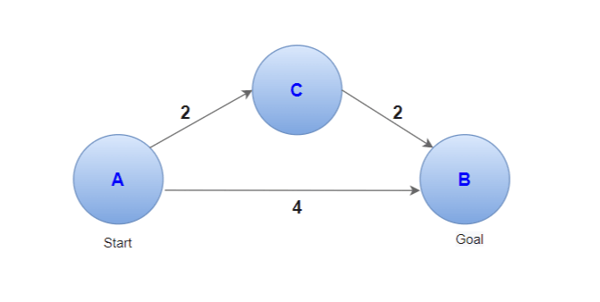Related Tags

ucs
algorithm
communitycreator

# What is a uniform cost search algorithm?

Alishba Ehsan

## Overview

Search algorithms are used to resolve search problems. For example, search for the shortest path between two given points, searching for a goal, and so on.

Suppose we want to move from point A to point B and there are two paths between these two points. Which path would you choose? These types of problems can be solved using search algorithms.

## Uniform Cost Search

Uniform Cost Search (UCS) is a type of uninformed searchblind search that performs a search based on the lowest path cost. UCS helps us find the path from the starting node to the goal node with the minimum path cost. Considering the scenario that we need to move from point A to point B, which path would you choose? A->C->B or A->B:Graph

The path cost of going from path A to path B is 5 and the path cost of path A to C to B is 4 (2+2). As UCS will consider the least path cost, that is, 4. Hence, A to C to B would be selected in terms of uniform cost search.

### Explanation

#### Concept:

• Frontier list Fringe or Open Listwill be based on the priority queue. Every new node will be added at the end of the list and the list will give priority to the least cost path.
• The node at the top of the frontier list will be added to the expand listNode to expand, which shows that this node is going to be explored in the next step. It will not repeat any node. If the node has already been explored, you can discard it.
• Explored listClosed List will be having the nodes list, which will be completely explored.

#### Algo:

1. Add the Starting node S in the frontier list with the path cost g(n) = 0 (starting point is at 0 path cost).
2. Add this node to the Explored list, as we only have a single node in the frontier list. If we have multiple nodes, then we will add that one node at the top of the frontier.
3. Now, explore this node by visiting all of its child nodes. After that, add this node to the Explored list, as it is now fully explored.
4. Check if the added node is the goal node or not. Stop if the goal node is found, or else move on to the next step.
5. Since new nodes are added to the frontier list, we need to compare and set the priority queue again, depending upon the priority, that is, the minimum path cost g(n).
6. Now, move to back to step 2 and repeat the steps until the goal node is not added to the explored list.

### Solutions:

• Actual path: This is obtained by the frontier list.
• Traversed path: This is obtained by the explored list.

### Example

Consider the following graph. Let the starting node be A and the goal node be G.

Finding path A to G using uniform cost search

Implementing UCS:

 Frontier List Expand List Explored List {(A,0)} A NULL 2. {(A-D, 3), (A-B, 5)} D {A} 3. {(A-B, 5), (A-D-E, 5), (A-D-F, 5)} B {A, D} 4. {(A-D-E, 5), (A-D-F, 5), (A-B-C, 6)} E {A, D, B} 5. {(A-D-F, 5), (A-B-C, 6), (A-D-E-B, 9)}*here B is already explored F {A, D, B, E} 6. {(A-B-C, 6), (A-D-F-G,8)} C {A, D, B, E, F} 7. {(A-D-F-G,8), (A-B-C-E,12), (A-B-C-G, 14)}*here E is already explored G {A, D, B, E, F, C} 8. {(A-D-F-G,8)} NULL {A, D, B, E, F, C, G} # GOAL Found!

Hence we get:

1. Actual path => A -- D -- F -- G , with Path Cost = 8.
2. Traversed path => A -- D -- B -- E -- F -- C -- G

### Evaluation

The uniform cost search algorithm can be evaluated by four of the following factors:

• Completeness: UCS is complete if the branching factor b is finite.
• Time complexity: The time complexity of UCS is exponential O(b(1+C/ε)), because every node is checked.
• Space completeness: The space complexity is also exponential O(b(1+C/ε)), because all the nodes are added to the list for comparisons of priority.
• Optimality: UCS gives the optimal solution or path to the goal node.

RELATED TAGS

ucs
algorithm
communitycreator

CONTRIBUTOR

Alishba Ehsan
RELATED COURSES

View all Courses

Keep Exploring

Learn in-demand tech skills in half the time